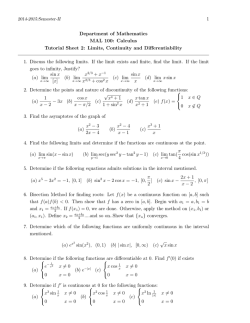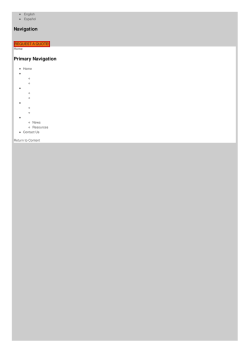# f(x, y) = 9 sin x siny ⇒ fx = 9 cosx siny, fy = 9 sin x cosy - WebAssign

```f (x, y) = 9 sin x sin y ⇒ fx = 9 cos x sin y, fy = 9 sin x cos y,
fxx = −9 sin x sin y, fxy = 9 cos x cos y, fyy = −9 sin x sin y. Here we have
−π < x < π and −π < y < π, so fx = 0 implies cos x = 0 or sin y = 0.
If cos x = 0 then x = − π2 or π2 , and if sin y = 0 then y = 0. Substituting
x = ± π2 into fy = 0 gives cos y = 0 ⇒ y = − π2 or π2 , and substituting
y = 0 into fy = 0 gives sin x = 0 ⇒ x = 0. Thus the critical points are
− π2 , ± π2 , π2 , ± π2 , and (0, 0).
D(0, 0) = −81 < 0 so (0, 0) is a saddle point.
D − π2 , ± π2 = D
π
, ± π2
2
fxx − π2 , − π2 = fxx
fxx − π2 , π2 = fxx
π π
,
2 2
π
, − π2
2
= 81 > 0 and
= −9 < 0 while
= 9 > 0, so f − π2 , − π2 = f
maxima and f − π2 , π2 = f
π
, − π2
2
π π
,
2 2
= 9 are local
= −9 are local minima.
8
6
4
2
0
–2
–4
–6
–8
–3
–2
–1
y
0
1
2
3
3
2
1
0
–1
x
–2
–3
```# 1 Department of Mathematics MAL 100: Calculus Tutorial Sheet 2# ukuhlolwa kwelizweloke komnyaka nomnyaka 2014 igreyidi 3